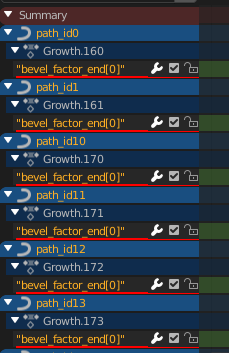# "bevel_factor_end" - keyframing with linear interpolation

Hi - I’m struggling with converting my keyframes to linear. I’ve done a lot of Googling, but none of the presented solutions have worked so far for me. I provided an extract of the relevant script below. Note that the “collection” is a collection with curve objects only.

The first part of the code works. I can create keyframes for all my objects but they default to Bezier. Now, I found a snippet (2nd part) that should convert the keyframes to manual. But, I think the issue is that with my keyframe insert method it doesn’t put this in the animation_data block?? (I verified that the created curve after the script indeed lists animation_data as ‘none’)

``````for ob in collection.all_objects:

for t in range(Sim_Duration+20):

if t==ob["start_time"]:
ob.data.bevel_factor_end = 0
ob.data.keyframe_insert(data_path="bevel_factor_end", index=-1,frame=t)
if t==ob["end_time"]:
ob.data.bevel_factor_end = 1
ob.data.keyframe_insert(data_path="bevel_factor_end", index=-1,frame=t)

fcurves = ob.animation_data.action.fcurves # here I get the error that a nonetype object has no attribute action
for fcurve in fcurves:
for kf in fcurve.keyframe_points:
kf.interpolation = 'CONSTANT'

type or paste code here
``````
1 Like

I also tried a different approach by explicitly creating fcurves via animation_data. Trouble here is that the data_path doesn’t seem to be recognised and it just creates a new property (?) for each curve and then appends an index:

``````
for ob in collection.all_objects:
ob.animation_data_create()
ob.animation_data.action = bpy.data.actions.new(name="Growth")
fcu=ob.animation_data.action.fcurves.new(data_path='bevel_factor_end')

for t in range(Sim_Duration+20):

if t==ob["start_time"]:
#ob.data.bevel_factor_end = 0
#ob.data.keyframe_insert(data_path="bevel_factor_end", index=-1,frame=t)
fcu.keyframe_points.co = t,0
if t==ob["end_time"]:
#ob.data.bevel_factor_end = 1
#ob.data.keyframe_insert(data_path="bevel_factor_end", index=-1,frame=t)
fcu.keyframe_points.co = t,1
``````Forgot to say that when I then add the 2nd piece of code, the interpolation is set correctly! It’s just that the keyframes don’t tie in with the actual variable/property that I want to animate!.

So, this is the “full” code that leads to what I want: change of interpolation to constant for all the keyframes:

``````for ob in collection.all_objects:
ob.animation_data_create()
ob.animation_data.action = bpy.data.actions.new(name="Growth")
fcu=ob.animation_data.action.fcurves.new(data_path='bevel_factor_end', index=-1)

for t in range(Sim_Duration+20):

if t==ob["start_time"]:
#ob.data.bevel_factor_end = 0
#ob.data.keyframe_insert(data_path="bevel_factor_end", index=-1,frame=t)
fcu.keyframe_points.co = t,0
if t==ob["end_time"]:
#ob.data.bevel_factor_end = 1
#ob.data.keyframe_insert(data_path="bevel_factor_end", index=-1,frame=t)
fcu.keyframe_points.co = t,1

fcurves = ob.animation_data.action.fcurves
for fcurve in fcurves:
for kf in fcurve.keyframe_points:
kf.interpolation = 'CONSTANT'
type or paste code here
``````

By the way, the above code does work with other properties like ‘scale’… it’s just that it doesn’t seem to recognize bevel_factor_end as a built in propery?

Any other ideas from anyone? I’m beginning to think it’s impossible with the current API? Hopefully I’m missing something though!

OK - so, seems I was wrong (happy to admit!) I managed to make it work. I’ll leave some code later on. But one of the issues was just stupidity on my part; one of the curves I had didn’t have any keyframes set (conditions not met) and hence I got an error message about action not being available.